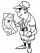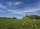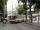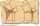# Bomber

Bomber flies 10 km at 600 km/h. At what horizontal distance from the target, must pilot drop the bomb to hit the target?

Don't care about air resistance and consider the gravitational acceleration g=9.81 m/s2.

Result

The pilot must drop the bomb on target before:  7525 m

#### Solution:

Leave us a comment of this math problem and its solution (i.e. if it is still somewhat unclear...):Be the first to comment!#### Following knowledge from mathematics are needed to solve this word math problem:

Looking for help with calculating roots of a quadratic equation? Do you want to convert length units?

## Next similar math problems:

1. Bike rideMarek rode a bike ride. In an hour, John followed him on the same route by car, at an average speed of 72 km/h, and in 20 minutes he drove him. Will he determine the length of the way that Marek took before John caught up with him, and at what speed did Ma
2. Tourist JirkaDistance between the points A and B is 13.5 km. Jirka went from point A to point B unknown speed and for an unknown period of time. Back to the point A went slower by 3 km/h which means that went 20 minutes more. How long Jirka took the return journey?
3. Distance problem 2A=(x,2x) B=(2x,1) Distance AB=√2, find value of x
4. Distance problemA=(x, x) B=(1,4) Distance AB=√5, find x;
5. Distance between 2 pointsFind the distance between the points (7, -9), (-1, -9)
6. Two chordsCalculate the length of chord AB and perpendicular chord BC to circle if AB is 4 cm from the center of the circle and BC 8 cm from the center of the circle.
7. TrainsFrom station 130 km away started passenger train and after 2.2 hours after the express train, which travels 37 km an hour more. Express train finish journey 7 minutes early. Calculate the average speed of this two trains.
8. Built-up areaJohn build up area 5 x 7 = 35 m2 with building with a wall thickness 30 cm. How many centimeters would have to subtract from thickness of the walls that built-up area fell by 9%?
9. Two cyclists 2At the same time, two cyclists left the towns A and B at constant speeds. The first one going from town A to town B, and the second one from town B to town A. At one point of the trip they met. After they met, the first cyclist arrived at town B in 36min,.
10. Faces diagonalsIf the diagonals of a cuboid are x, y, and z (wall diagonals or three faces) respectively than find the volume of a cuboid. Solve for x=1.2, y=1.7, z=1.45
11. CuboidCuboid with edge a=16 cm and body diagonal u=45 cm has volume V=11840 cm3. Calculate the length of the other edges.
12. Rectangle SSPerimeter of a rectangle is 268 cm and its diagonal is 99.3 cm. Determine the dimensions of the rectangle.
13. TrolleybusTrolleybus line No. 206 measured 24 km. If the trolleybus goes faster by 5 km/h, the way there and back would is shorter by 33 minutes. Calculate the trolleybus speed and how much time it takes a return trip.
14. Euclid3Calculate height and sides of the right triangle, if one leg is a = 81 cm and section of hypotenuse adjacent to the second leg cb = 39 cm.
15. AbyssStone was pushed into the abyss: 2 seconds after we heard hitting the bottom. How deep is the abyss (neglecting air resistance)? (gravitational acceleration g = 9.81 m/s2 and the speed of sound in air v = 343 m/s)
16. Space diagonalThe space diagonal of a cube is 129.91 mm. Find the lateral area, surface area and the volume of the cube.
17. The touristThe tourist traveled 78km in 3 hours. Part of the journey went on foot at 6km/h, the rest of the trip by bus at 30km/h. How long did he walk?